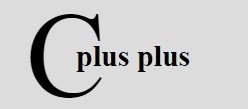# C++ for while do-while Loop

The iteration (for, while, and do-while loop) statements allows a set of instruction to be performed repeatedly until a certain condition is fulfilled. The iteration statements are also called loops or looping statements. C++ provides three kinds of loops :

• for loop
• while loop
• do-while loop

All three loop constructs of C++ repeat a set of statements as long as a specified condition remains true. This specified condition is generally referred to as a loop control. For all three loop statements, a true condition is any nonzero value. A zero value indicates a false condition.

### Loop Parts

Every loops has its elements that control and govern its execution. Generally, a loop has four elements that have different purposes. These elements are as :

• Initialization Expression(s)
• Test Expression
• Update Expression(s)
• Loop's Body

Let's discuss these parts of a loop one by one.

### Initialization Expression(s)

Before entering in a loop, its control variable(s) must be initialized. The initialization of the control variable(s) takes place under initialization expression(s). The initialization expression(s) give(s) the loop variable(s) their first value(s). The initialization expression(s) is executed only once, in the beginning of the loop.

### Test Expression

The test expression is an expression whose truth value decides whether the loop-body will be executed or not. If the test expression evaluates to true i.e., 1, the loop-body gets executed, otherwise the loop is terminated.

In an entry-controlled loop, the test-expression is evaluated before exiting from the loop. In C++, the for loop and while loop are entry-controlled loops and do-while loop is exit-controlled loop.

### Update Expression(s)

The update expression(s) change the value(s) of loop variable(s). The update expression(s) is executed; at the end of the loop after the loop-body is executed.

### Loop's Body

The statements that are executed repeatedly (as long as the test-expression is nonzero) from the body of the loop. In an entry-controlled loop, first test-expression is evaluated and if it is nonzero, the body-of-the-loop is executed; if the test-expression evaluates to be zero, the loop is terminated. In an exit-controlled loop, the body-of-the-loop is executed first and then the test-expression is evaluated. If it evaluates to be zero, the loop is terminated otherwise repeated. Now let's discuss the C++ loops: for, while and do-while.

## C++ for Loop

The for loop is the easiest to understand of the C++ loops. All its loop-control elements are gathered in one place (on the top of the loop), while in the other loop construction of C++, they (top-control elements) are scattered about the program.

### C++ for Loop Syntax

The general form (syntax) of the for loop statement is :

```for(initialization expression(s); test-expression; update expression(s))
{
body-of-the-loop ;
}```

### C++ for Loop Example

The following example program illustrates the use of for statement :

```/* C++ Iteration Statements - C++ for Loop */

#include<iostream.h>
#include<conio.h>
void main()
{
clrscr();
int i;
for(i=1; i<=10; i++)
{
cout<<i<<" ";
}
getch();
}```

When the above program is compile and executed, it will produce the following output: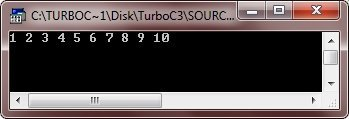The following lines explain the working of the above given for loop program :

• Firstly, initialization expression is executed, i.e., i=1 which gives the first value 1 to variable i.
• Then, the test expression is evaluated i.e., i <= 10 which results into true i.e., 1.
• Since, the test expression is true, the body-of-the-loop i.e., cout << i << " ";
is executed which prints the current value of i then a single space.
• After executing the loop-body, the update expression i.e., i++ is executed which increments the value of i. (After first execution of the loop, the value of i becomes 2 after the execution of i++, since initially i was 1).
• AFter the update expression is executed, the test-expression is again evaluated. If it is true the sequence is repeated from the step no 3, otherwise the loop terminates.

Note - Use for loop when you have to repeat a block of statements specific number of times.

## C++ Infinite Loop

Although any loop statement can be used to create an infinite loop (endless loop) yet for is traditionally used for this purpose. An infinite for loop can be created by omitting the test-expression as shown below :

```for(i=25; ; i--)
{
cout << "This loop runs forever..!!" ;
}```

Similarly, the following for loop is also an infinite loop :

```for( ; ; )
{
cout << "I will not stop..!!" ;
}```

Here is an example of infinite loop in C++

```/* C++ Loops - C++ Infinite Loop */

#include<iostream.h>
#include<conio.h>
void main()
{
clrscr();
for( ; ; )
{
cout<<"This loop will run forever..!!";
}
getch();
}```

When the conditional expression is absent, it is assumed to be true. You may have an initialization and increment expression, but C++ programmers more commonly use the for(;;) construct to signify an infinite loop. If you try to run the above C++ program, then the output window will come out like this, printing continuously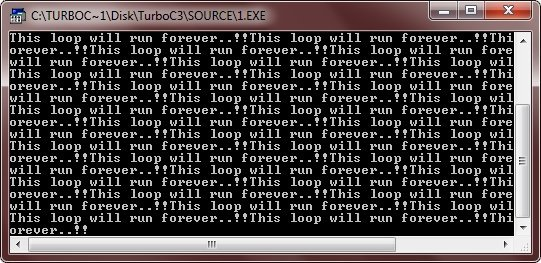NOTE - You can terminate an infinite loop by pressing Ctrl+C keys. Let's take another program, prints stars infinite times

```/* C++ Loops - C++ Infinite Loop */

#include<iostream.h>
#include<conio.h>
void main()
{
clrscr();
for(;;)
{
cout<<"*  ";
}
getch();
}```

After running this C++ program, output window will look like this, printing stars infinite times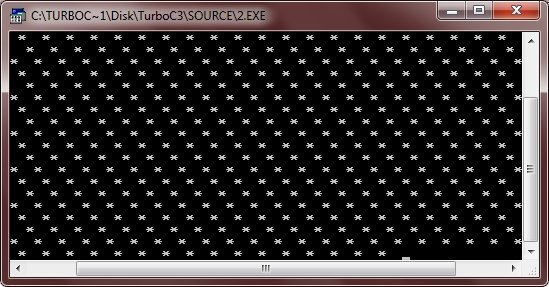### C++ Empty Loop

If a loop does not contain any statement in its loop-body, it is said to be an empty loop. In such cases, a C++ loop contains an empty statement i.e., a null statement. Following for loop is an empty loop:

`for(i=20; (i); i--) ;`

See, the body of the above for loop contains just (a null statement). It is an empty loop. An empty for loop has its applications in pointer manipulations where you need to increment or decrement pointer position without doing anything else.

Time delay loops are often used in programs. The following code shows how to create one by using for :

`for(t = 0; t < 3000; t++) ;`

That means if you put a semicolon after for's parenthesis it repeats only for counting the control variable. And if put a block of statements after such a loop, then it is not part of for loop. For example, consider the following :

```for(i=0; i<10; i++) ;
{
cout << "i = " << i << endl;
}```

The semicolon after the for loop ends the loop there only. And then, the below i.e, is not body of the for loop.

## C++ while Loop

The second loop available in C++ is the while loop. The while loop is an empty-controlled loop.

### C++ while Loop Syntax

Following is the syntax of the while loop :

```while(expression)
{
loop-body ;
}```

where the loop-body may contain a single statement, a compound statement or an empty statement. The loop iterates while the expression evaluates to true. When the expression becomes false, the program control passes to the line after the loop-body code.

In a while loop, a loop control variable should be initialized before the loop begins as an uninitialized variable can be used in the expression. The loop variable should be updated inside the body-of-the-while.

### C++ while Loop Example

Following example program illustrates the working of a while loop :

```/* C++ Iteration Statements - C++ while Loop */

#include<iostream.h>
#include<conio.h>
void main()
{
clrscr();
unsigned long num, fact=1;
cout<<"Enter a number: ";
cin>>num;
while(num)
{
fact = fact*num;
num--;
}
cout<<"The factorial of the number is "<<fact;
getch();
}```

When the above program compile and executed, it will produce the following output:The above program inputs an integer num. Then as long as num is nonzero (according to while (num)) the loop-body iterates i.e., fact is multiplied with num and the result is stored back in fact, followed by the decrement of num. Again the test-expression (num) is evaluated : if it is true, the loop repeated otherwise terminated.

## C++ do-while Loop

Unlike the for and while loops, the do-while is an exit-controlled loop i.e., it evaluates its test-expression at the bottom of the loop after executing its loop-body statements. This means that a do-while loop always executes at least once.

In the other two loops for and while, the test-expression is evaluated at the beginning of the loop i.e., before executing the loop-body. If the test-expression evaluates to false for the first time itself, the loop is never executed. But in some situations, it is wanted that the loop-body is executed at least once, no matter what the initial state of the test-expression is. In such cases, the do-while loop is the obvious choice.

### C++ do-while Loop Syntax

The syntax of the do-while loop is:

```do
{
statement ;
}while(test-expression);```

The braces { } are not necessary when the loop-body contains a single statement. The following do-while loop prints all upper-case letters :

```char ch = 'A' ;
do
{
cout << "\n" << ch ;
ch++;
}while(ch <= 'Z');```

The above code prints characters from 'A' onwards until the condition ch <= 'Z' becomes false.

### C++ do-while Loop Example

The most common use of the do-while loop is in menu selection routine, where the menu is flashed at least once. Then depending upon the user's response it is either repeated or terminated.

The following example program is a menu selection program. Following program display a menu regarding rectangle operations and perform according to the user's response :

```/* C++ Iteration Statements - C++ do-while Loop */

#include<iostream.h>
#include<conio.h>
#include<math.h>
#include<process.h>
void main()
{
clrscr();
char ch, ch1;
float l, b, peri, area, diag;
cout<<"Rectangle Menu";
cout<<"\n 1. Area";
cout<<"\n 2. Perimeter";
cout<<"\n 3. Diagonal";
cout<<"\n 4. Exit\n";
cout<<"\nEnter your choice: ";
do
{
cin>>ch;
if(ch == '1' || ch == '2' || ch == '3')
{
cout<<"Enter length & breadth: ";
cin>>l>>b;
}
switch(ch)
{
case '1' : area = l * b ;
cout<<"Area = "<<area;
break ;
case '2' : peri = 2 * (l + b);
cout<<"Perimeter = "<<peri;
break;
case '3' : diag = sqrt((l * l) + (b * b));
cout<<"Diagonal = "<<diag;
break;
case '4' : cout<<"Breaking..Press a key..";
getch();
exit(1);
default : cout<<"Wrong choice !!!!";
cout<<"\nEnter a valid one";
break;
}     //end of switch
cout<<"\nWant to enter more (y/n) ? ";
cin>>ch1;
if(ch1 == 'y' || ch1 == 'Y')
cout<<"Again enter choice (1-4): ";
}while(ch1 == 'y' || ch1 == 'Y') ;      //end of DO-WHILE loop
getch();
}```

When the above program is compile and executed, it will produce the following output: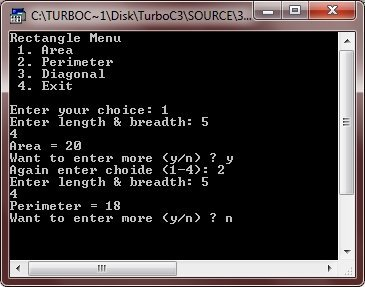## C++ Nested Loops

A loop may contain another loop in its body. This form of a loop is called nested loop. But in a nested loop, the inner loop must terminate before the outer loop. The following is an example of a nested loop.

```/* C++ Iteration Statements - C++ Nested Loops */

#include<iostream.h>
#include<conio.h>
void main()
{
clrscr();
int i, j;
for(i=0; i<15; i++)
{
for(j=0; j<=i; j++)
{
cout<<"* ";
}
cout<<"\n";
}
getch();
}```

When the above program is compile and executed it will produce the following output: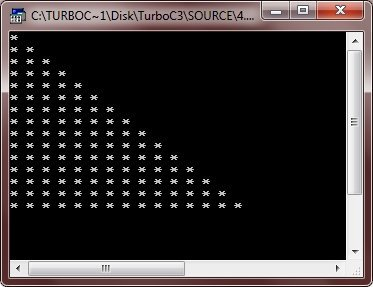Here is one more program, demonstrating nested loop in C++

```/* C++ Loops Program */

#include<iostream.h>
#include<conio.h>
void main()
{
clrscr();
int num, temp, i, j;
cout<<"Enter a number: ";
cin>>num;
for(i=0; i<10; i++)
{
num++;
temp=num;
for(j=0; j<=i; j++)
{
cout<<num<<" ";
num++;
}
cout<<"\n";
num=temp;
}
getch();
}```

Below is the sample run of the above C++ program:### More Examples

Here are some more examples listed, that you may like:

Tools
Calculator

Quick Links
Signup - Login - Give Online Test